﻿ 埋地金属管道杂散电流腐蚀影响因素的研究 Influence Factors of Stray-Current Corrosion of Buried Metal Pipeline

Applied Physics
Vol. 09  No. 05 ( 2019 ), Article ID: 30343 , 9 pages
10.12677/APP.2019.95030

Influence Factors of Stray-Current Corrosion of Buried Metal Pipeline

Fanxing Meng, Yaping Zhang*, Jialei Zhang

College of Science, China University of Petroleum (East China), Qingdao Shandong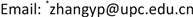Received: May 2nd, 2019; accepted: May 15th, 2019; published: May 22nd, 2019ABSTRACT

Buried pipeline leakage due to corrosion will cause immeasurable impact. The corrosion effect of stray current will seriously affect the normal use of buried pipeline, and it is necessary to study the factors affecting stray current corrosion to put forward reasonable and effective anti-corrosion measures. There is rare news research about the size of the stray current in different applied voltages and under the different conditions of different soil. Through the laboratory simulation, the recent reference electrode method is adapted to pipe-to-soil potential measurements, and the corrosion behavior of pipeline is studied in different applied voltages and under different soil environments. The results showed that the use of coating protection can suppress the corrosion to a certain extent. Within a certain content range, with the increase of NaCl mass fraction, the ground potential showed an increasing trend. In a certain depth range, with the increase of the buried depth, the ground potential gradually decreases; with the increase of applied voltage, the ground potential increases gradually.

Keywords:Buried Pipeline, Scattered Current, Impact Factors, Pipe Voltage1. 引言

2. 实验部分

2.1. 腐蚀原理

1) 当金属铁处于酸性电解质中，发生的是析氢腐蚀，主要反应如下：

$4{\text{H}}_{2}\text{O}+4{\text{e}}^{-}\to 4{\text{OH}}^{-}+2{\text{H}}_{2}↑$

2) 当金属铁处于碱性电解质中，发生的是吸氧腐蚀，主要反应如下：

${\text{O}}_{2}+2{\text{H}}_{2}\text{O}+4{\text{e}}^{-}\to 4{\text{OH}}^{-}$Figure 1. Schematic diagram of corrosion perforationFigure 2. Schematic diagram of current distribution in subway system

${I}_{3}=\frac{1}{12}{I}_{1}\frac{R}{{R}_{g}}{L}^{2}$ (1)

${R}_{g}$ ：每公里钢轨的对地电阻(过渡电阻)，单位是 $\Omega \cdot \text{km}$

R：每公里钢轨阻抗，单位是 $\Omega \cdot \text{km}$

${I}_{1}$ ：机车的牵引电流，单位是A；

${I}_{3}$ ：腐蚀电流，单位是A；

L：变电站间距，单位是km。

$\Delta W=kQ=k{I}_{3}\Delta t$ (2)

$\Delta W$ ：金属在 $\Delta t$ 时间内的析出量，kg；

Q：在 $\Delta t$ 时间内流过金属的电量，C；

k：金属的电化学当量；

${I}_{3}$ ：腐蚀电流，A；

$\Delta t$ ：通电时间，s。

2.2. 实验方法与内容

2.2.1. 实验装置设计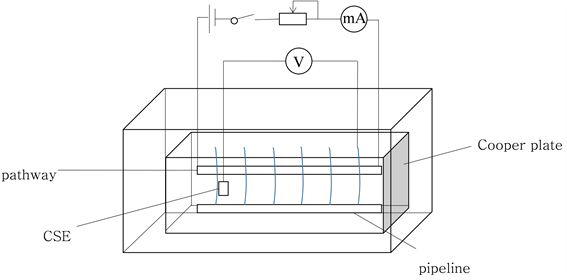Figure 3. Diagram of experimental device

2.2.2. 实验材料Table 1. Chemical composition of 304 L pipeline steel

2.2.3. 腐蚀测试

3. 结果与讨论

3.1. 防护涂层对管地电位的影响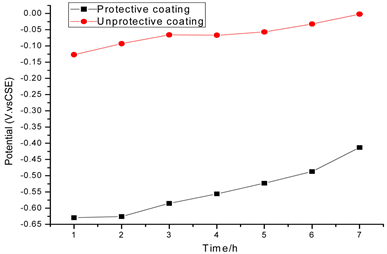Figure 4. Comparison of ground voltage of metal tubes with or without protective coating at different times

3.2. NaCl含量对管地电压的影响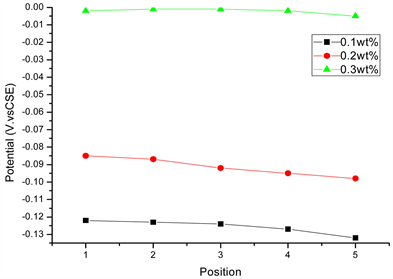Figure 5. Ground voltage of metal pipeline with protective coating at different concentrations

$2{\text{CI}}^{-}+{\text{Fe}}^{2\text{+}}\to {\text{FeCI}}_{2}$ (3)

3.3. 埋地深度对管地电位的影响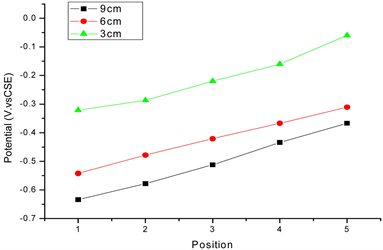Figure 6. Ground potential measured at different epths at the same concentration

3.4. 外加电压对管地电位的影响Figure 7. Ground potential measured under different applied voltages in test points

4. 结论

Influence Factors of Stray-Current Corrosion of Buried Metal Pipeline[J]. 应用物理, 2019, 09(05): 250-258. https://doi.org/10.12677/APP.2019.95030

1. 1. 高延宁, 王凤军, 王志刚, 等. 长输管线杂散电流腐蚀检测与防护[J]. 油气田地面工程, 2002, 21(3): 25-26.

2. 2. 张瑞. 地铁车站杂散电流腐蚀防护措施研究[J]. 低碳世界, 2018(5): 259-260.

3. 3. 李长春. 埋地钢质管道直流杂散电流腐蚀机理及影响因素研究[J]. 中国新技术新产品, 2017(1): 50-51.

4. 4. Lucca, G. (2015) Estimating Stray Current Interference from DC Traction Lines on Buried Pipelines by Means of a Monte Carlo Algorithm. Electrical Engineering, 97, 277-286. https://doi.org/10.1007/s00202-015-0333-6

5. 5. 李志宏. 埋地钢制管道杂散电流腐蚀检测参数的选择及测量方法研究[J]. 轻工科技, 2015(1): 95-96.

6. 6. 钱建华, 闫永贵, 李威力. 城市轨道交通杂散电流对埋地管线腐蚀的影响[J]. 腐蚀科学与防护技术, 2009, 21(3): 250-251.

7. 7. 汪洋, 张亚萍, 韩秀虹, 等. 减小来自阴极保护装置杂散电流干扰的实验探究[J]. 现代物理, 2015, 5(3): 65-71.

8. 8. 朱王晶. 阴极极化对590高强钢及其焊接件氢脆敏感性影响的研究[D]: [硕士学位论文]. 青岛: 青岛科技大学, 2014.

9. 9. 封琼, 张亚萍, 余豪, 于濂清, 李焰. 土壤电阻率对埋地管道杂散电流腐蚀影响的研究进展[J]. 应用物理, 2015, 5(10): 123-130.

10. 10. Zhang, Y.P., Feng, Q., Yu, L.Q., et al. (2019) Numerical Modelling of Buried Pipelines under DC Stray Current Corrosion. Journal of Electrochemical Science and Engineering, 9, 125-134. https://doi.org/10.5599/jese.567

11. 11. 李可. 金属管道的电化学腐蚀与防护[J]. 油气田地面工程, 2013, 32(5): 118.

12. 12. 大庆石油管理局油田建设设计研究院. 钢质管道及储罐防腐蚀工程设计规范: SYJ 7-84[M]. 北京: 石油工业出版社, 1984.

13. 13. 封琼, 张亚萍, 汪洋, 等. 基于埋地金属管道杂散电流的腐蚀与防护[J]. 腐蚀与防护, 2017, 38(2): 91-95.

14. 14. 胡士信. 阴极保护工程手册[M]. 北京: 化学工业出版社, 1999.

15. 15. 徐承伟, 李树新, 张一峰, 等. 一种可应用于冻土区的便携式Cu/CuSO4参比电极[J]. 全面腐蚀控制, 2014, 28(7): 45-48.

16. 16. 张曼曼. 埋地管道防腐层破损对阴极保护参数影响规律研究[D]: [硕士学位论文]. 北京: 中国民航大学, 2016.

17. 17. 何文寿, 刘阳春, 何进宇. 宁夏不同类型盐渍化土壤水溶盐含量与其电导率的关系[J]. 干旱地区农业研究, 2010, 28(1): 111-116.

18. 18. 吴月茹, 王维真, 王海兵, 等. 采用新电导率指标分析土壤盐分变化规律[J]. 土壤学报, 2011, 48(4): 869-873.

19. 19. Lü, G.C., Xu, C.C., Lü, Y.M., et al. (2008) The Enrichment of Chloride Anion in the Oc-cluded Cell and Its Effect on Stress Corrosion Crack of 304 Stainless Steel in Low Chloride Concentration Solution. Chinese Journal of Chemical Engineering, 16, 646-649. https://doi.org/10.1016/S1004-9541(08)60135-4

20. 20. Huang, H.L., Pan, Z.Q., Guo, X.P., et al. (2014) Effects of Direct Current Electric Field on Corrosion Behaviour of Copper, CI− Ion Migration Behaviour and Dendrites Growth under Thin Electrolyte Layer. Transactions of Nonferrous Metals Society of China, 24, 285-291. https://doi.org/10.1016/S1003-6326(14)63059-4

21. 21. 耿健, 丁庆军, 孙家瑛, 等. 杂散电流影响下氯离子向混凝土内部的传输特征[J]. 建筑材料学报, 2010, 13(1): 121-124.

22. 22. 朱瑶宏, 邹玉生, 耿健, 等. 杂散电流对氯离子在混凝土内部迁移过程的影响[J]. 武汉理工大学学报, 2012, 34(7): 32-36.

23. 23. 应蓉蓉, 林玉锁, 段光明. 土壤环境保护标准体系框架研究[J]. 环境保护, 2015, 43(7): 60-63.

24. NOTES

*通讯作者。